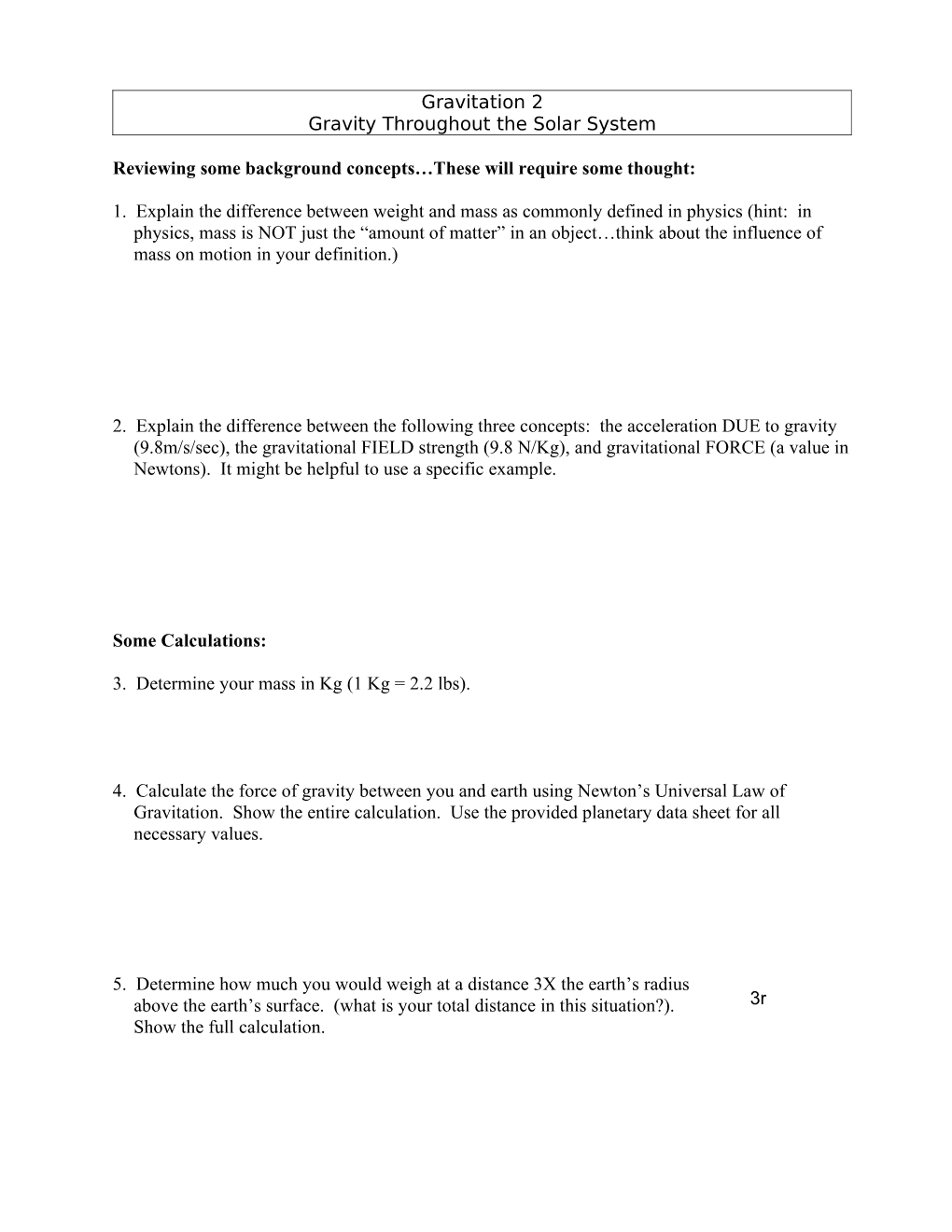# Determining Your Weight Throughout the Solar SystemGravitation 2

Gravity Throughout the Solar System

Reviewing some background concepts…These will require some thought:

1. Explain the difference between weight and mass as commonly defined in physics (hint: in physics, mass is NOT just the “amount of matter” in an object…think about the influence of mass on motion in your definition.)

2. Explain the difference between the following three concepts: the acceleration DUE to gravity (9.8m/s/sec), the gravitational FIELD strength (9.8 N/Kg), and gravitational FORCE (a value in Newtons). It might be helpful to use a specific example.

Some Calculations:

3. Determine your mass in Kg (1 Kg = 2.2 lbs).

4. Calculate the force of gravity between you and earth using Newton’s Universal Law of Gravitation. Show the entire calculation. Use the provided planetary data sheet for all necessary values.

5. Determine how much you would weigh at a distance 3X the earth’s radius above the earth’s surface. (what is your total distance in this situation?). Show the full calculation.

6. The space shuttle often orbits at a distance of about 200 miles above the earth’s surface. Convert this distance into meters (1 mi = 1609 m) and calculate the force of the earth on an 80 Kg astronaut at this point in space. Some of your best friends believe that astronauts on the shuttle float because “there is no gravity in space”. Does your calculation here support that view? What percentage of one’s surface weight is present 200 miles above the earth’s surface?

7. Determine how much you would weigh at the surface of the moon. (i.e. what is the force of gravity between you and the moon?). Show the full calculation.

8. Determine how much you would weigh at the surface of Jupiter. Show the full calculation.

9. Determine how much you would weigh at the surface of the sun.

10. Determine the force of gravity between the earth and the moon.

11. Imagine that the sun was to shrink without losing mass (we will discuss why some stars collapse very soon in class). What would happen to the forces of gravity on objects at the sun’s surface? Explain your reasoning fully. What would happen to the force of gravity between the earth and sun? Explain.

12. If the sun were to shrink to the size of the earth without losing any mass, how much would a 1 kg object weigh at its surface? Show your calculation clearly.

13. If the sun were to shrink to the size of the earth without losing mass, would the force of gravity of the sun acting on the earth and other planets change? Explain.

14. Describe the difference between free fall motion, projectile motion, and orbital motion. What do each of these have in common?